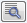# 4.2.3 Iteration Parameters

### Iteration Parameters

The locked setting options of this dialog section are not required for RF-CONCRETE Members: The FE division allowing for a fine control of the convergence behavior is used for nonlinear calculations.

Click thebutton to open the FE Mesh dialog box where you can adjust the global target length of elements of the FE mesh and the division specifications for members.

Iteration Parameters

You can control the iteration process with the parameters in this dialog section.

Max. number of iterations per load increment

The iteration process strongly depends on the cross-section shape, the structural system, and the loading. Thus, the number of iterations required to reach the break-off limits is exposed to strong fluctuations. The preset value of 50 iterations is sufficient for most practical applications but can be adjusted, if necessary.

Damping of the change in stiffness between two iteration steps

The program determines the difference in stiffness on a node in the course of two successive iteration steps. The Damping factor represents the part of the stiffness difference that is considered for the new stiffness applied in the subsequent iteration step. By reducing the stiffness changes between two iteration steps, it is possible to counteract the calculation's oscillation.

The higher the damping factor, the smaller the damping's influence. If the factor is equal to 1, the damping does not affect the iterative calculation.

Break Limits ε1 / ε2 / ε3

The break-off limits can be adjusted depending on purpose and function: Even if relatively roughly defined break limits (ε1 = ε2 ≤ 0.01) lead to sufficiently accurate results when calculating according to linear static analysis (beam deformations in SLS, for example), it is nevertheless recommended to refine the tolerances used for stability analyses (ε1 = ε2 ≤ 0.001). Example 3 in chapter 9.3 illustrates the effect clearly.

With the break limit ε3, you can additionally control the deformation change. This criterion observes how the size of the maximum deformation changes. The specified damping factor is also taken into account.

The loading can be gradually applied in order to avoid or attenuate an abrupt stiffness change within the individual finite elements ("adapting" the system to the loading). The aim is to avoid the generation of major stiffness changes during an iteration. When the loading is applied step-by-step, it is possible in the iteration step of a load increment to always fall back on the corresponding final stiffness of the element from the previous load increment.

Number of load increments

This input field determines the number of individual load increments for the nonlinear calculation.